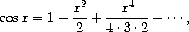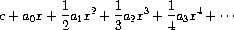# Power series represented as infinite streams

In section 2.5.3 we saw how to implement a polynomial arithmetic system representing polynomials as lists of terms. In a similar way, we can work with power series, such asrepresented as infinite streams. We will represent the series `a₀ + a₁x + a₂x² + a₃x³ + ···` as the stream whose elements are the coefficients `a₀, a₁, a₂, a₃, ...` .

a. The integral of the series `a₀ + a₁x + a₂x² + a₃x³ + ···` is the serieswhere `c` is any constant. Define a procedure `integrate-series` that takes as input a stream `a₀, a₁, a₂, ...` representing a power series and returns the stream `a₀, (1/2)a₁, (1/3)a₂, ...` of coefficients of the non-constant terms of the integral of the series. (Since the result has no constant term, it doesn't represent a power series; when we use `integrate-series` , we will `cons` on the appropriate constant.)

b. The function `x → eˣ` is its own derivative. This implies that `eˣ` and the integral of `eˣ` are the same series, except for the constant term, which is `e⁰ = 1` . Accordingly, we can generate the series for `eˣ` as

``````(define exp-series
(cons-stream 1 (integrate-series exp-series)))
``````

Show how to generate the series for sine and cosine, starting from the facts that the derivative of sine is cosine and the derivative of cosine is the negative of sine:

``````(define cosine-series
(cons-stream 1 <??>))
(define sine-series
(cons-stream 0 <??>))
``````